﻿ NLVM 6 - 8 - Algebra Manipulatives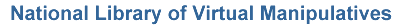Algebra Balance Scales – Solve simple linear equations using a balance beam representation.Algebra Balance Scales - Negatives – Solve simple linear equations using a balance beam representation.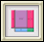Algebra Tiles – Visualize multiplying and factoring algebraic expressions using tiles.Base Blocks – Illustrate addition and subtraction in a variety of bases.Block Patterns – Analyze sequences of figures using pictures, tables, plots, and graphs.Coin Problem – Use deduction to find the counterfeit coin.Factor Tree – Factor numbers using a tree diagram.Fifteen Puzzle – Solve this virtual version of the classical fifteen puzzle by arranging its tiles.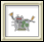Function Machine – Explore the concept of functions by putting values into this machine and observing its output.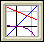Function Transformations – Explore how simple transformations affect the graph of a function.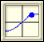Grapher – A tool for graphing and exploring functions.Line Plotter – Practice drawing lines through a given point having a specified slope.Pattern Blocks – Use six common geometric shapes to build patterns and solve problems.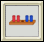Peg Puzzle – Win this game by moving the pegs on the left past the pegs on the right.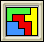Pentominoes – Use the 12 pentomino combinations to solve problems.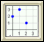Point Plotter – Practice plotting ordered pairs on a graph.Polyominoes – Build and compare characteristics of biominoes, triominoes, quadrominoes, etc.Space Blocks – Create and discover patterns using three dimensional blocks.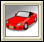Stick or Switch – Investigate probabilities of sticking with a decision, or switching.Towers of Hanoi – Solve the tower problem and test your theory by varying the number of disks.Triominoes – Manipulate the puzzle pieces and find multiple solutions.